Courses

# Test: CAT Quant 2018 (Shift - 2)

## 34 Questions MCQ Test Quantitative Aptitude (Quant) | Test: CAT Quant 2018 (Shift - 2)

Description
This mock test of Test: CAT Quant 2018 (Shift - 2) for CAT helps you for every CAT entrance exam. This contains 34 Multiple Choice Questions for CAT Test: CAT Quant 2018 (Shift - 2) (mcq) to study with solutions a complete question bank. The solved questions answers in this Test: CAT Quant 2018 (Shift - 2) quiz give you a good mix of easy questions and tough questions. CAT students definitely take this Test: CAT Quant 2018 (Shift - 2) exercise for a better result in the exam. You can find other Test: CAT Quant 2018 (Shift - 2) extra questions, long questions & short questions for CAT on EduRev as well by searching above.
QUESTION: 1

### A water tank has inlets of two types A and B. All inlets of type A when open bring in water at the same rate. All inlets of type B, when open, bring in water at the same rate. The empty tank is completely filled in 30 minutes if 10 inlets of type A and 45 inlets of type B are open and in 1 hour if 8 inlets of type A and 18 inlets of type B are open. In how many minutes will the empty tank get completely filled if 7 inlets of type A and 27 inlets of type B are open?

Solution: Let the efficiency of type A pipe be 'a' and the efficiency of type B be 'b'.

In the first case, 10 type A and 45 type B pipes fill the tank in 30 mins.

So, the capacity of the tank = 1/2 (10a + 45b)…..(i)

In the second case, 8 type A and 18 type B pipes fill the tank in 1 hour.

So, the capacity of the tank = (8a + 18b)…. (ii)

Equating (i) and (ii), we get

10a + 45b = 16a + 36b

=>6a=9b

From (ii), capacity of the tank = (8a + 18b) = (8a + 12a) = 20a

In the third case, 7 type A arid 27 type B pipes fill the tank.

Net efficiency = (7a + 27b) = (7a + 18a) = 25a

Time taken = 20a/25a hour = 48 minutes.

Hence, 48 is the correct answer.

QUESTION: 2

### Let f(x) = max (5x, 52 - 2x2), where x is any positive real number. Then the minimum possible value of f(x)

Solution: The minimum value of the function will occur when the expressions inside the function are equal.

So, 5x = 52 - 2x2

or, 2x2 + 5x - 52 = 0

on solving, we get x = 4 or 13/-2

But, it is given that x is a positive number.

So, x = 4

and the minimum value = 5*4 = 20

Hence, 20 is the correct answer.

QUESTION: 3

### Points A, P, Q and B lie on the same line such that P, Q and B are, respectively, 100 km, 200 km and 300 km away from A. Cars 1 and 2 leave A at the same time and move towards B. Simultaneously, car 3 leaves B and moves towards A. Car 3 meets car 1 at Q, and car 2 at P. If each car is moving in uniform speed then the ratio of the speed of car 2 to that of car 1 is

Solution: Car 3 meets car 1 at Q, which is 200 km from A.

Therefore, at the time of their meeting car 1 must have travelled 200 km and car 3 must have travelled 100 km.

As the time is same, ratio of speed of, car 1 to speed of car 3 = 2:1.

Car 3 meets car 2 at P, which is 100 km from A.

Therefore, at the time of their meeting car 2 must have travelled 100 km and car 3 must have travelled 200 km.

As the time is same, ratio of speed of car 2 to speed of car 3 = 1 : 2.

Speed of car 1: speed of car 3 = 2:1

And speed of car 2: speed of car 3 = 1: 2

So, speed of car 1: speed of car 2: speed of car 3 = 4:1: 2

Hence, option D is the correct answer.

QUESTION: 4

The smallest integer n such that n3 - 11n2 + 32n - 28 > 0 is

Solution: We can see that at n = 2, n3 — 11n2 + 32n - 28 = 0 i.e. (n-2) is a factor of n3 - 11n2 + 32n - 28 (n3 - 11n2 + 32n - 28n) /2 = n2 - 9n +14

We can further factorize n2-9n+14 as (n-2) (n-7).

(n3 - 11n2 + 32n - 28 = (n - 2)2(n - 7)

=>n3- 11n2 + 32n - 28 > 0

=> (n - 2)2(n - 7) > 0

Therefore, we can say that n-7>0

Hence, nmin = 8

QUESTION: 5

The scores of Amal and Bimal in an examination are in the ratio 11:14. After an appeal, their scores increase by the same amount and their new scores are in the ratio 47: 56. The ratio of Bimal's new score to that of his original score is

Solution: Let the score of Amal and Bimal be 11 k and 14k

Let the scores be increased by x

So, after increment, AmaTs score = 11 k + x and Bimal's score = 14k + x

According to the question,

11k – x = 47

14k + x = 56

On solving, we get x = 42/9 k

Ratio of Bimal's new score to his original score

= (14k +x) /14k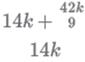= 168k/14*9k

= 4/3

Hence, option A is the correct answer.

QUESTION: 6

How many two-digit numbers, with a non-zero digit in the units place, are there which are more than thrice the number formed by interchanging the positions of its digits?

Solution: Let 'ab' be the two digit number. Where b ≠ 0.

We will get number 'ba' after interchanging its digit.

It is given that 10a+b > 3*(10b + a)

7a > 29b

If b = 1, then a = {5,6,7,8,9}

If b = 2, then a = {9}

If b = 3, then no value of 'a' is possible. Hence, we can say that thereare a total of 6 such numbers.

QUESTION: 7

For two sets A and B, let AΔB denote the set of elements which belong to A or B but not both. If P = {1,2,3,4}, Q = {2,3,5,6,}, R = {1,3,7,8,9}, S = {2,4,9,10}, then the number of elements in (PΔQ) Δ(RΔS) is

Solution: P = {1,2,3,4} and Q = {2,3,5,6,}

PΔQ ={1,4, 5, 6}

R = {1,3,7,8,9} and S = {2,4,9, 10}

RΔS = {1,2, 3,4,7, 8,10}

(PΔQ) Δ(RΔS) = {2, 3, 5, 6,7, 8,10}

Thus, there are 7 elements in (PΔQ)A(RΔS).

hence, 7 is the correct answer.

QUESTION: 8

A parallelogram ABCD has area 48 sq.cm. If the length of CD is 8 cm and that of AD is s cm, then which one of the following is necessarily true?

Solution: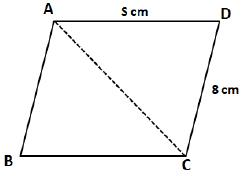we can see that area of parallelogram ABCD = 2*Area of triangle ACD

48 = 2*Area of triangle ACD

Area of triangle ACD = 24

(1/2) * CD * DA * sin ADC = 24

we know that sinϴ ≤ 1, Hence, we can say that AD ≥ 6

=> s ≥ 6

QUESTION: 9

A 20% ethanol solution is mixed with another ethanol solution, say, S of unknown concentration in the proportion 1:3 by volume. This mixture is then mixed with an equal volume of 20% ethanol solution. If the resultant mixture is a 31.25% ethanol solution, then the unknown concentration of S is

Solution: Let the volume of the first and the second solution be 100 and 300.

When they are mixed, quantity of ethanol in the mixture

= (20 + 300S)

Let this solution be mixed with equal volume i.e. 400 of third solution in which the strength of ethanol is 20%.

So, the quantity of ethanol in the final solution

= (20 + 300S + 80) = (300S + 100)

It is given that, 31.25% of 800 = (300S + 100)

or, 300S + 100 = 250

or S = 1/2 = 50%

Hence, 50 is the correct answer.

QUESTION: 10

In a tournament, there are 43 junior level and 51 senior level participants. Each pair of juniors play one match. Each pair of seniors play one match. There is no junior versus senior match. The number of girl versus girl matches in junior level is 153, while the number of boy versus boy matches in senior level is 276. The number of matches a boy plays against a girl is

Solution: In a tournament, there are 43 junior level and 51 senior level participants.

Let 'n' be the number of girls on junior level. It is given that the number of girl versus girl matches in junior level is 1530.

=>nC2 = 153

=> n (n-1)/2 = 153

=>n (n-1) = 306

=> n2 – n-306 = 0

=> (n+17) (n-18) =0

=>n=18 (rejecting n=-17)

Therefore, number of boys on junior level = 43 -18 = 25.

Let 'm' be the number of boys on senior level. It is given that the number of boy versus boy matches in senior level is 276

=> mC2 = 276

m = 24

Therefore, number of girls on senior level = 51 - 24 = 27.

Hence, the number of matches a boy plays against a girl = 18*25+24*27 = 1098

QUESTION: 11

A chord of length 5 cm subtends an angle of 60° at the centre of a circle. The length, in cm, of a chord that subtends an angle of 120° at the centre of the same circle is

Solution: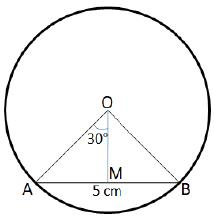We are given that AB = 5 cm and

Let us draw OM such that OM ⊥AB.

In right angle triangle AMO

sin30° = AM/AO

=> AO = 2*AM = 2*2.5 = 5 cm.

Therefore, we can say that the radius of the circle = 5 cm.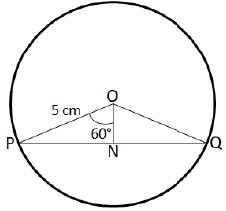In right angle triangle PNO,

Sin60◦ = PN/PO

=>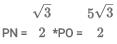Therefore, PQ = 2*PN = 5√3 cm

QUESTION: 12

Let a1, a2...a52 be positive integers such that a1< a2< ... < a52. Suppose, their arithmetic mean is one less than arithmetic mean of a2, a3… a52. If a52= 100, then the largest possible value of a1

Solution: Let 'x' be the average of all 52 positive integers a1, a2...a52.

a1 + a2 + a3 + ... + a52 = 52x ... (1)

Therefore, average of a2, a3, ....a52 = x+1

a2 + a3 + a4 + ... a52 = 51 (x+1)... (2)

From equation (1) and (2), we can say that

a1 + 51(x + 1) = 52x

a1 = x - 51.

We have to find out the largest possible value of a1.a1 will be maximum when 'x' is maximum.

(x+1) is the average of terms a2, a3 ...a52. We know that a2< a3< ... < a52 and a52 = 100.

Therefore, (x+1) will be maximum when each term is maximum possible. If a52 = 100, then a52 = 99, a50 = 98 ends so on.

a2 = 100 + (51 -1)*(-1) = 50.

Hence, a2 + a3 + a4 + ... + a52 = 50+51+...+99+100 = 51 (x+1)

51 * (50 + 100)/2 = 51(x + 1)

x = 74

Therefore, the largest possible value of a1 = x - 51 =74- 51 = 23.

QUESTION: 13

The value of the sum 7 x 11 + 11 x 15 + 15 x 19 + ...+95 x 99 is

Solution: S = 7x11 +11 x15 + 15x19 + ...+ 95 x 99

Nth term of the series can be written as Tn = (4n + 3) * (4n + 7)

Last term, (4n+3) = 95 i.e. n = 23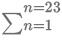(4n + 3)*(4n + 7)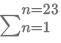16n2 + 40n + 21

16* (23*24*47)/6 + 40 * (23 * 24)/2 + 21* 23

= 80707

QUESTION: 14

If N and x are positive integers such that NN = 2160 and N2 + 2N is an integral multiple of 2X, then the largest possible x is

Solution:

It is given that NN = 2160.

We can rewrite the equation as NN = (25)160/5 = 3232

=> N = 32

N2 + 2N = 322 + 232 = 210 + 232 = 210 * (1 + 222)

Hence, we can say that N2 + 2N can be divided by 210

Therefore, Xmax = 10

QUESTION: 15

A tank is emptied everyday at a fixed time point. Immediately thereafter, either pump A or pump B or both start working until the tank is full. On Monday, An alone completed filling the tank at 8 pm. On Tuesday, B alone completed filling the tank at 6 pm. On Wednesday, A alone worked till 5 pm, and then B worked alone from 5 pm to 7 pm, to fill the tank. At what time was the tank filled on Thursday if both pumps were used simultaneously all along?

Solution: Let't' pm be the time when the tank is emptied every day. Let ’a' and. 'b' be the liters/hr filled by pump A and pump B respectively.

On Monday, An alone completed filling the tank at 8 pm. Therefore, we can say that pump A worked for (8 -1) hours. Hence, the volume of the tank = a*(8 -1) liters.

Similarly, on Tuesday, B alone completed filling the tank at 6 pm. Therefore, we can say that pump B worked for (6 -1) hours. Hence, the volume of the tank = b*(6 -1) liters.

On Wednesday, An alone worked till 5 pm, and then B worked alone from 5 pm to 7 pm, to fill the tank. Therefore, we can say that pump A worked for (5 -t) hours and pump B worked for 2 hours. Hence, the volume of the tank = a*(5 - t) +2b liters.

We can say that a*(8-t) = b*(6-t) = a*(5- t) + 2b

a*(8 -1) = a*(5 -1) + 2b

3a = 2b ... (1)

a*(8 -1) = b*(6-1)

Using equation (1), we can say that

a * (8 - t) = 3a/2 * (6 -t)

t = 2

Therefore, we can say that the tank gets emptied at 2 pm daily. We can see that A takes 6 hours and pump B takes 4 hours alone.

Hence, working together both can fill the tank in = frac{6*4}{6+4} = 2.4 hours or 2 hours and 24 minutes.

The pumps started filling the tank at 2:00 pm. Hence, the tank will be filled by 4:24 pm.

QUESTION: 16

The arithmetic mean of x, y and z is 80, and that of x, y, z, u and v is 75, where u=(x+y)/2 and v=(y+z)/2. If x > z, then the minimum possible value of x is

Solution: Given that the arithmetic mean of x, y and z is 80.

=> x + y + z /3 = 80

=> x + y + z = 240 ... (1)

Also, x+y+z+v+u/5 = 75

x+y+z+v+u/5 = 75

=> x + y + z + v + u = 375

Substituting values from equation (1),

=>v + u = 135

It is given that u=(x+y)/2 and v=(y+z)/2

=> (x + y)/2 + (y + z)/2 = 135

=> x + 2y + z = 270

=> y = 30 (Since x + y + z = 240)

Therefore, we can say that x + z = 240 - y = 210. We are also given that x > z,

Hence, Xmin = 210/2 = 105.

QUESTION: 17

Points A and B are 150 km apart. Cars 1 and 2 travel from A to B, but car 2 starts from A when car 1 is already 20 km away from A. Each car travels at a speed of 100 kmph for the first 50 km, at 50 kmph for the next 50 km, and at 25 kmph for the last 50 km. The distance, in km, between car 2 and B when car 1 reaches B is

Solution: Time taken to cover first 50 km at 100 km/hr = 1/2 hr.

Time taken to cover second 50 km at 50 km/hr = 1 hr.

Time taken to cover last 50 km at 25 km/hr = 2 hr.

When car 2 starts, car 1 has already covered 20 km.

So, time taken by car 1 to reach B after car 1 starts = total time - time required to travel first 20 km

= 3 hr 30 min -12 min = 3 hr 18 min

Distance travelled by car 2 = (50 + 50 + 45) = 145 km

Distance from B = (150 -145) km = 5 km

Hence, 5 is the correct answer.

QUESTION: 18

If the sum of squares of two numbers is 97, then which one of the following cannot be their product?

Solution:

Let 'a' and 'b' are those two numbers.

=> a2 + b2 = 97

=>a2 + b2 = 2ab = 97 — 2 ab

=> (a – b) 2 = 97 - 2ab

we know that (a2 – b2) ≥ 0

=>97-2ab ≥ 0

=>ab ≤ 48.5

Hence, ab ≠ 64.

Therefore, option D is the correct answer.

QUESTION: 19

The area of a rectangle and the square of its perimeter are in the ratio 1:25. Then the lengths of the shorter and longer sides of the rectangle are in the ratio

Solution: Let 'a' and 'b' be the length of sides of the rectangle, (a > b)

Area of the rectangle = a*b

Perimeter of the rectangle = 2*(a+b)

=>a*b/(2*(a + b))2 = 1/25

=> 25ab = 4(a + b)2

=> 4a2 - 17ab + 4b2 = 0

=> (4a - b)(a - 4b) = 0

=> a = 4b or b/4

We initially assumed that a > b, therefore a ≠ 4.

Hence, a = 4b

=> b: a = 1: 4

QUESTION: 20

On a triangle ABC, a circle with diameter BC is drawn, intersecting AB and AC at points P and Q, respectively. If the lengths of AB, AC, and CP are 30 cm, 25 cm, and 20 cm respectively, then the length of BQ, in cm, is

Solution: Let us draw the diagram according to the available information.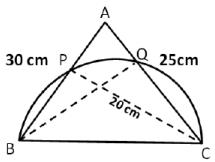We can see that triangle BPC and BQC are inscribed inside a semicircle. Hence, we can say that

Therefore, we can say that BQ ⊥ AC and CP ⊥ AB.

In triangle ABC,

Area of triangle = (1/2)*Base*Height = (1/2)*AB*CP = (1/2)*AC*BQ

=> BQ = AB *CP/AC = 30 * 20/25 = 24 cm.

QUESTION: 21

A triangle ABC has area 32 sq units and its side BC, of length 8 units, lies on the line x = 4. Then the shortest possible distance between A and the point (0,0) is

Solution: We know that area of the triangle = 32 sq. units, BC = 8 units

Therefore, the height of the perpendicular drawn from point A to BC = 2*32/8 = 8 units.

Let us draw a possible diagram of the given triangle.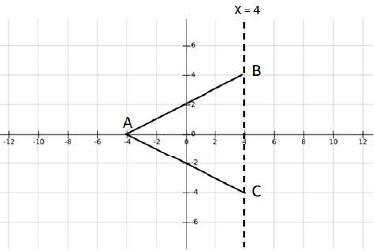We can see that if A coincide with (-4, 0) then the distance between A and (0, 0) = 4 units.

If we move the triangle up or down keeping the base BC on x = 4, then point A will move away from origin as vertical distance will come into factor whereas horizontal distance will remain as 4 units.

Hence, we can say that minimum distance between A and origin (0, 0) = 4 units.

Positive Mark: 3

Negative Mark: 0

QUESTION: 22

From a rectangle ABCD of area 768 sq cm, a semicircular part with diameter AB and area 72π sq cm is removed. The perimeter of the leftover portion, in cm, is

Solution: Area of the semicircle with AB as a diameter = 1/2 * π *(AB2/ 4)

=>1/2 * π *(AB2/ 4) = 72 * π

=> AB = 24cm

Given that area of the rectangle ABCD = 768 sq.cm

=>AB*BC = 768

=>BC = 32 cm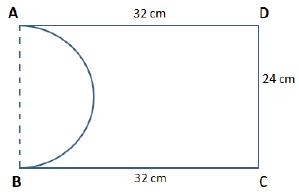We can see that the perimeter of the remaining shape = AD + DC + BC + Arc(AB)

=> 32+24+32+π * 24/2

=> 88 + 12π

QUESTION: 23

If A = {62n - 35n - 1}, where n= 1,2,3,... and B = {35(n-1)}, where n = 1,2,3,... then which of the following is true?

Solution: If we carefully observe set A, then we find that 62n - 35n - 1 is divisible by 35. So, set A contains multiples of 35. However, not all the multiples of 35 are there in set A, for different values of n.

For n = 1, the value is 0, for n = 2, the value is 1225 which is the 35th multiple of 3.

If we observe set B, it consists of all the multiples of 35 including 0.

So, we can say that every member of set A will be in B while every member of set B will not necessarilybe in set A.

Hence, option A is the correct answer.

QUESTION: 24

The smallest integer n for which 4n> 1719 holds, is closest to

Solution: 4n> 1719

=> 16n/2> 1719

Therefore, we can say that n/2 > 19

n > 38

Hence, option D is the correct answer.

QUESTION: 25

A jar contains a mixture of 175 ml water and 700 ml alcohol. Gopal takes out 10% of the mixture and substitutes it by water of the same amount. The process is repeated once again. The percentage ff water in the mixture is now

Solution: Final quantity of alcohol in the mixture = 700/(700 + 175) * 90/(100 )2 * [700 + 175] = 567 ml

Therefore, final quantity of water in the mixture = 875 - 567 = 308 ml

Hence, we can say that the percentage of water in the mixture = 308/875 x 100 = 35.2%

QUESTION: 26

If a and b are integers such that 2x2- ax + 2 > 0 and x2 - bx + 8 ≥ 0 for all real numbers x, then the largest possible value of 2a - 6b is

Solution:

Let f(x) = 2x2 - ax + 2. We can see that f(x) is a quadratic function.

For, f(x) > 0, Discriminant (D) < />

=> (-a)2 – 4*2*2< />

=> (a-4)(a+4) < />

=> a ε (-4,4)

Therefore, integer values that 'a' can take = {-3, -2, -1,0,1,2,3}

Let g(x) = x2- bx + 8. We can see that g(x) is also a quadratic function.

For, g(x)≥0, Discriminant (D) ≤ 0

=> (-b)2– 4*8* 1 < />

=>(b - √32 ) (b + √32 ) < />

=> b ε(-√32 ,√32 )

Therefore, integer values that 'b' can take = {-5, -4, -3, -2, -1,0,1,2,-3,4,5}

We have to find out the largest possible value of 2a - 6b.

The largest possible value will occur when 'a' is maximum and 'b' is minimum.

amax = 3, bmin = -5

Therefore, the largest possible value of 2a - 6b – 2*3 – 6*(-5) = 36.

QUESTION: 27

1/log2100 – 1/log4100 + 1/log5100 – 1/log10100 + 1/log20100 – 1/log25100 + 1/log50100?

Solution: We know that 1/logab = logxa/logxb

Therefore, we can say that 1/log2100 = log102/ log10100

=>1/Iog2100-1/Iog4100 – 1/Iog5100 - log10100 + 1/Iog20100 -1/Iog25100 + 1/Iog50100

=> log102/log10100 - log104/log10100 + log105/log10100 - log1010/log10100 + log1020/log10100 - log1025/log10100 + log1050/log10100

We know that log10100 = 2

=> 1/2 * [log102 -log104+ log105-log1010+ log1020-log1025+ log1050]

=>1/2 *[log10 (2*5*20*50)/(4 *10 *25)]

=> 1/2 * [log1010]

=>½

QUESTION: 28

If p3 = q4 = r5 = s6, then the value of logs(pqr) is equal to

Solution:

Given that, p3 = q4 = r5 = s6

p3=s6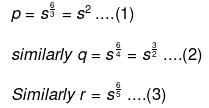=> logs(pqr)

By substituting value of p, q, and r from equation (1), (2) and (3)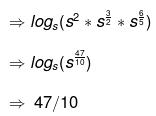QUESTION: 29

There are two drums, each containing a mixture of paints A and B. In drum 1, A and B are in the ratio 18:7. The mixtures from drums 1 and 2 are mixed in the ratio 3: 4 and in this final mixture, A and B are in the ratio 13:7. In drum 2, then A and B were in the ratio

Solution: It is given that in drum 1, A and B are in the ratio 18:7.

Let us assume that in drum 2, A and B are in the ratio x: 1.

It is given that drums 1 and 2 are mixed in the ratio 3:4 and in this final mixture, A and B are in the ratio 13:7.

By equating concentration of A

3 * 18/ (18 + 7 + 4) * x/(x + 1)

=>3 + 4=13/ (13 + 7)

=>54/25 + 4x/x+1 = 91/20

=> 4x/(x+1)= 239/100

=>x= 239/161

Therefore, we can say that in drum, 2, A and B are in the ratio 239/161 :1 or 239:161.

QUESTION: 30

Ramesh and Ganesh can together complete a work in 16 days. After seven days of working together, Ramesh got sick and his efficiency fell by 30%. As a result, they completed the work in 17 days instead of 16 days. If Ganesh had worked alone after Ramesh got sick, in how many days would he have completed the remaining work?

Solution: Let 'R' and 'G' be the amount of work that Ramesh and Ganesh can complete in a day.

It is given that they can together complete a work in 16 days. Hence, total amount of work = 16(R+G)... (1)

For first 7 days both of them worked together. From 8th day, Ramesh worked at 70% of his original efficiency whereas Ganesh worked at his original efficiency. It took them 17 days to finish the same work, i.e., Ramesh worked at 70% of his original efficiency for 10 days.

=> 16(R+G) = 7(R+G)+10(0.7R+G)

=> 16(R+G) = 14R+17G

=> R = 0.5G ... (2)

Total amount of work left when Ramesh got sick = 16(R+G) - 7(R+G) = 9(R+G) = 9(0.5+G) = 13.5G

Therefore, time taken by Ganesh to complete the remaining work = 13.5/G =13.5 days.

QUESTION: 31

Gopal borrows Rs. X from Ankit at 8% annual interest. He then adds Rs. Y of his own money and lends Rs. X+Y to Ishan at 10% annual interest. At the end of the year, after returning Ankit's dues, the net interest retained by Gopal is the same as that accrued to Ankit. On the other hand, had Gopal lent Rs. X+2Y to Ishan at 10%, then the net interest retained by him would have increased by Rs. 150. If all interests are compounded annually, then find the value of X + Y.

Solution: Amount of interest paid by Ishan to Gopal if the borrowed amount is Rs. (X+Y) = 10/100 * (X + Y) =0.1 (X+Y)

Gopal also borrowed Rs. X from Ankit at 8% per annum. Therefore, he has to return Ankit Rs. 0.08X as the interest amount on borrowed sum.

Hence, the interest retained by gopal = 0.1 (X+Y) - 0.08X = 0.02X + 0.1 Y ... (1)

It is given that the net interest retained by Gopal is the same as that accrued to Ankit.

Therefore, 0.08X = 0.02X + 0.1 Y

=>X = (5/3)Y ...(2)

Amount of interest paid by Ishan to Gopal if the borrowed amount is Rs. (X+2Y) = 10/100 * (X + 2Y) = 0.1X+0.2Y

In this case the amount of interest retained by Gopal = 0.1 X+0.2Y - 0.08X = 0.02X + 0.2Y ... (3)

It is given that the interest retained by Gopal increased by Rs. 150 in the second case.

=>(0.02X + 0.2Y) - (0.02X + 0.1 Y) = 150

=> Y = Rs. 1500

By substituting value of Y in equation (2), we can say that X = Rs. 2500

Therefore, (X+Y) = Rs. 4000.

QUESTION: 32

Thestrength of a salt solution is p% if 100 ml of the solution contains p grams of salt. If three salt solutions A, B, C are mixed in the proportion 1: 2:3, then the resulting solution has strength 20%. If instead the proportion is 3:2:1, then the resulting solution has strength 30%. A fourth solution, D, is produced by mixing B and C in the ratio 2: 7. The ratio of the strength of D to that of A is

Solution: Let 'a', 'b' and 'c' be the concentration of salt in solutions A, B and C respectively.

It is given that three salt solutions A, B, C are mixed in the proportion 1:2: 3, then the resulting solution has strength 20%.

=> a + 2b + 3c/1+2+3 = 20

=> a + 2b + 3c = 120….. (1)

If instead the proportion is 3 : 2 :1, then the resulting solution has strength 30%.

=> 3a + 2b + 3c/1+2+3 = 30

=>3a + 2b + c = 180 ... (2)

From equation (1) and (2), we can say that

=> b + 2c = 45

=> b = 45 – 2c

Also, on subtracting (1) from (2), we get

a - c = 30

=> a = 30 + c

In solution D, B and C are mixed in the ratio 2:7

So, the concentration of salt in D = 2b + 7c/9 = 90 - 4c + 7c /9 = 90 + 3c/9

Required ratio = 90 + 3c/9a = 90 + 3c/9(30+c) = 1 : 3

Hence, option B is the correct answer.

QUESTION: 33

On a long stretch of east-west road, A and B are two points such that B is 350 km west of A. One car starts from A and another from B at the same time. If they move towards each other, then they meet after 1 hour. If they both move towards east, then they meet in 7 hrs. The difference between their speeds, in km per hour, is

Solution: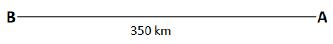Let 'a' and 'b' be the speed (in km/hr) of cars starting from both A and B respectively.

If they both move in east direction, then B will catch A if and only if b > a.

Relative speed of both the cars when they move in east direction = (b - a) km/hr

It takes them 7 hours to meet. I.e., they travel 350 km in 7 hours with a relative speed of (b-a) km/hr.

Hence, (b-a) = 350/7 = 50 km/hr.

QUESTION: 34

Let t1, t2... be real numbers such that t1 + t2 + ... + tn = 2n2 + 9n + 13, for every positive integer n ≥ 2. If tk = 103, then k equals

Solution: It is given that t1 + t2 + ... + tn = 2n2 + 9n + 13, for every positive integer n ≥ 2.

We can say that t1 + t2 + ... + tk = 2k2 + 9k + 13 ... (1)

Replacing k by (k-1) we can say that

t1 + t2 + …… + tk—1 = 2(k - 1)2 + 9(k - 1) +13 ... (2).

On subtracting equation (2) from equation (1)

=>tk = 2k2 + 9k+13- 2(k -1)2 + 9(k - 1) + 13

=> 103 = 4k + 7

k =24